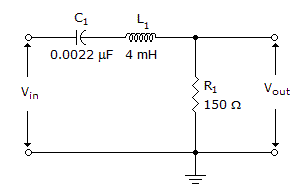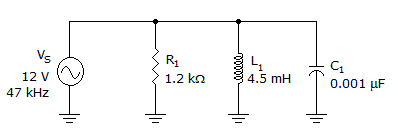# Electronics - RLC Circuits and Resonance

### Exercise :: RLC Circuits and Resonance - General Questions

26.What is the bandwidth in the given circuit. If the winding resistance of the coil is 12?

 A. 6.4 kHz B. 47.2 kHz C. 53.7 kHz D. 60.2 kHz

Explanation:

No answer description available for this question. Let us discuss.

27.

At any resonant frequency, what net voltage is measured across the two series reactive components?

 A. Applied voltage B. Reactive voltage C. Zero voltage D. VL + VC voltage

Explanation:

No answer description available for this question. Let us discuss.

28.

If the bandwidth of a filter increases:

 A. Q decreases B. the roll-off rate increases C. the half-power frequency decreases D. the center frequency decreases

Explanation:

No answer description available for this question. Let us discuss.

29.

Series RLC impedance or voltage totals must always be calculated by

 A. adding values vectorially B. graphing the angles C. multiplying the values D. subtracting the values

Explanation:

No answer description available for this question. Let us discuss.

30.What is the current through the capacitor in the given circuit?

 A. 3.5 mA B. 5.5 mA C. 9 mA D. 11.4 mA